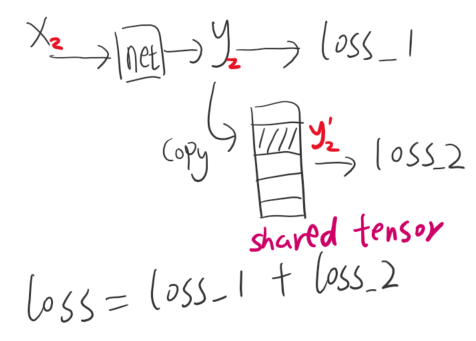# A problem about shared tensor, the growth of computation graph and loss calculation

I have a shared tensor used in different epochs, so I need to shared_tensor = tensor(require_grad).detach() , or I have to set loss.background( retain_graph=True ) because of the dependency of the shared_tensor. When I set retain_graph=True, the graph will grow during training.

The problem is that the one part in loss is based on the shared _tensor. For example, Loss = loss_a + loss_b, loss_b is from the shared_tensor, but since shared_tensor requires no grad (from tensor.detach() ), loss_b cannot be used to update the network in back propagation, right?

How to fix it? What’s the correct way to save the shared_tensor and calculate related loss?code:
yi=modelA(xi)
loss_1 = L1(yi,0)

yi’ = yi (by detach() or clone())
loss_2 = L1(torch.op([y1’, y2’…]))

loss = loss_1 + loss_2
loss.backward()

Can you be more precise? what do you refer when you say that the tensor is shared in different epochs?.

Anyway, if you have two models lets say model1 and model2, you can always replace one of the parameters of model2 with the same parameter of model1. In C code it would be the pointer of the parameter. You can easily do that:

``````import torch
a=torch.zeros((10,))
b=a
b=1
print(a)#you will see that a has been modified also
``````

Assume batch_size =1,
the input is xi, we get yi after the net (modelA) and store yi into a shared tensor yi’,
For example, the figure shows the example of input i=2.
If the input is x3, then we get y3 and then y3’. So the tensor [y1’, y2’, y3’…] is used in different epochs.

If y2’= y2.clone(), then y2’ also requires grad, so the shared tensor needs grad, if have to set loss.backward(retain_graph=True), or get "trying to backward through the graph a second time, but the buffers have already been freed. ". But if retrain_graph = True, the graph will grow continually to be too big.

if y2’= y2.detach(), there’s no the above problem. but loss_2 will not require grad, so network cannot be updated with loss_2.

Can I combine loss_1 and loss_2 together, and call backward() once? Or I should loss_1.backward() and loss_2.backward()?

I cannot see the figure.

The tensor [y1’.y2’…] how do you use it?. Please provide the algorithm or a depiction.

The 1st epoch:
input x1.
y1’ = model(x1), and store y1’. (y1’ requires grad)
calculate loss2 = L1(torch.op(y1’, y2’…)).
loss2.backward().

The next epoch:
input x2.
y2’ = model(x2), and store y2’. (y2’ requires grad)
calculate loss2 = L1(torch.op(y1’, y2’…)).

In the 2nd epoch, only y2’ need grad, y1’ and y3’ are constant results. so I should
set y1’.require_grad = False after the 1st epoch, after optimizer.step()?

ah okei. Yes, if y1’ is constant in the next epoch just convert it to a tensor that does not require grad, because if not it will try to go under a graph that has been released and will raise error.